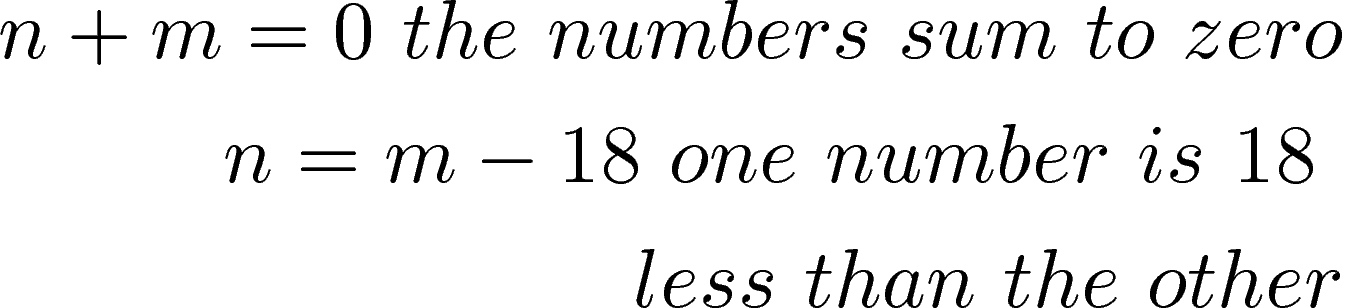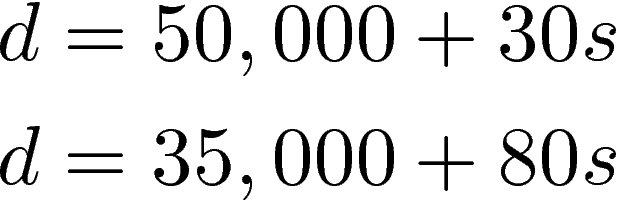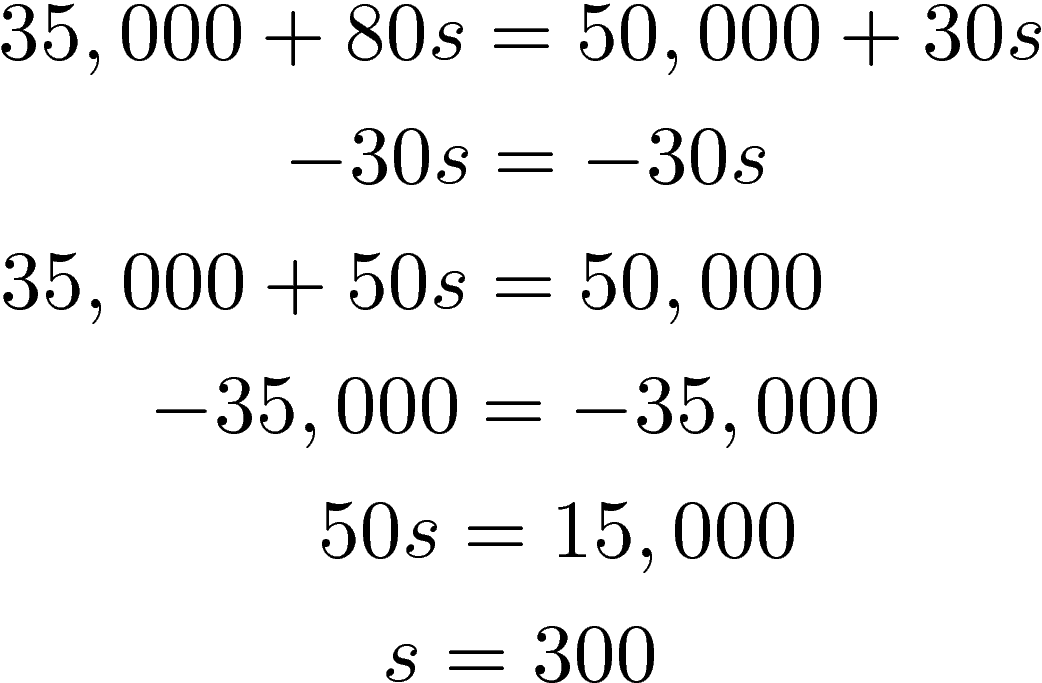# Solving a System of Equations with Direct Translation

In this post, we will look at two simple problems that require us to solve for a system of equations. Recall that a system of equations involves two or morevariables that must be solved. With each problem, we will use the direct translation to set up the problem so that it can be solved.

Direct Translation

Direct translation involves reading a problem and translating it into a system of equations. In order to do this, you must consider the following steps

1. Determine what you want to know
2. Assigned variables to what you want to know
3. Setup the system of equations
4. Solve the system

Example `1

Below is an example  followed by a step-by-step breakdown

The sum of two numbers is zero. One number is 18 less than the other. Find the numbers.

Step 1: We want to know what the two numbers are

Step 2: n = first number & m =  second number

Step 3: Set up systemSolving this is simple we know n = m – 18 so we plug this into the first equation n + m = 0  and solve for m.Now that we now m we can solve for n in the second equationThe answer is m = 9 and n = -9. If you add these together they would come to zero and meet the criteria for the problem.

Example 2

Below is a second example involving a decision for salary options.

Dan has been offered two options for his salary as a salesman. Option A would pay him \$50,000 plus \$30 for each sale he closes. Option B would pay him \$35,000 plus \$80 for each sale he closes. How many sales before the salaries are equal

Step 1: We want to know when the salaries are equal based on sales

Step 2: d =  Dan’s salary & s = number of sales

Step 3: Set up systemTo solve this problem we can simply substitute d  for one of the salaries as shown belowYou can check to see if this answer is correct yourself. In order for the two salaries to equal each other Dan would need to sale 300 units. After 300 units option B is more lucrative. Deciding which salary option to take would probably depend on how many sales Dan expects to make in a year.

Conclusion

Algebraic concepts can move beyond theoretical ideas and rearrange numbers to practical applications. This post showed how even something as obscure as a system of equations can actually be used to make financial decisions.